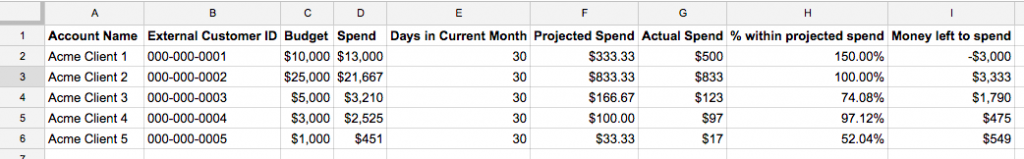As a small business agency, one of the hardest things you’ll have to do is to manage your clients AdWords budgets. Hopefully this tool will help your job easier. Start by creating a new spreadsheet in Google Drive, this will be where we store all of our work.To start, add five columns: Account name, External Client ID, Budget, Spend, Days in Current Month. Here we can enter all of necessary information to get started. The Account Nickname that you gave it, their AdWords Client ID, The amount of money to spend this month and the amount of money they’ve spent so far this month.

First, let’s set up the number of days in the current month. You can use this formula: =DAY(DATE(YEAR(today()),MONTH(today())+1,0)). For June, this will return the value 30. It uses the today function in spreadsheets, so it’s automatically updated every month.

Next, let’s figure out how much money we need to spend. Let’s create a new column and call it Projected Spend. Here we’ll divide the budget by the total number of days in the month. You can use the formula =cX/eX. Just replace the X with the row number that you’re on. For example, if your client wants to spend $10,000 for June, we know the spend needed would be$333.33 per day.

In column D we’ll record how much the client has spent for the month so far. This can be automated, but that’s for another day. For this practice, let’s assume that it’s June 6th and the client has spent $3,000.00. Let’s go ahead and create another column called Actual Spend Per Day. Here we can simply divide the total spend in column D by the number of days elapsed in the month so far. Since we don’t want to change this by hand daily, let’s use a formula. That formula will be =day(date(year(today()),month(today()), day(today()))). According to this column, the client is spending an average of$500.00. At this pace they’ll spend \$15,000 in June and spend all of their money in 20 days instead of 30. In another post, I’ll share how you can control this programmatically.

Another measure that we’ll want to measure is how close the actual spend is to the projected spend. Let’s add another column and call it % within projected spend. Here we are going to divide the actual spend by the projected spend. Here our formula tells us that we’re over our required spend by 150%.

If you manage a large number of accounts, this will give you a top level view of how each account is performing at any given time.

## What can this can this do for me?

You could extend this Google sheet in a few ways:

• First you could write a Google AdWords script that automates pulling in your account spend for the month every hour.
• You could also automate campaigns to automatically pause if the account reaches a certain threshold to prevent auto pays on a credit card.
• You could also extend the spreadsheet further to automatically manage your campaign budgets, keyword bids and day-parting bids to better control your accounts.